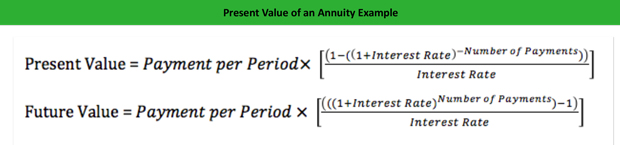# What is an Ordinary Annuity?

Definition: An ordinary annuity is a series of equal payments are paid at the end of each period for a defined amount of time.

## What Does Ordinary Annuity Mean?

What is the definition of ordinary annuity? The concept of an ordinary annuity is rather simple. It’s a stream of payments that do not change from period to period each occurring at the end of each period over a specific amount of time.

Ordinary annuities are often associated with the issuance of bonds with specific terms regarding the bond interest rate and the time to maturity. The bond terms allow us to determine the present value and the future value of the annuity payment. These calculations are useful when determining the true cash flows received or paid over the life of the annuity.

How do we calculate the value of an annuity? We can determine the present and future value of any annuity by using the following two equations:Let’s look at an example of each of these.

## Example

Let’s keep things simple and assume you receive an annuity payment of \$2,000 each year for 10 years. As you receive the annuity payments at the end of each year, you decide to save and invest the cash into a safe investment earning 4%. To better understand the true cash value of this annuity, let’s determine the present and future value:Based on the calculations above, it’s easy to determine the cash flow growth over the ten year term of the annuity. If all of annuity payments are saved and invested, the current cash flow that’s worth \$16,221 will grow to \$24,012 upon maturity of the annuity.

## Summary Definition

Define Ordinary Annuities: Annuity means a consistent set of payments paid at the end of each period over a defined amount of time.